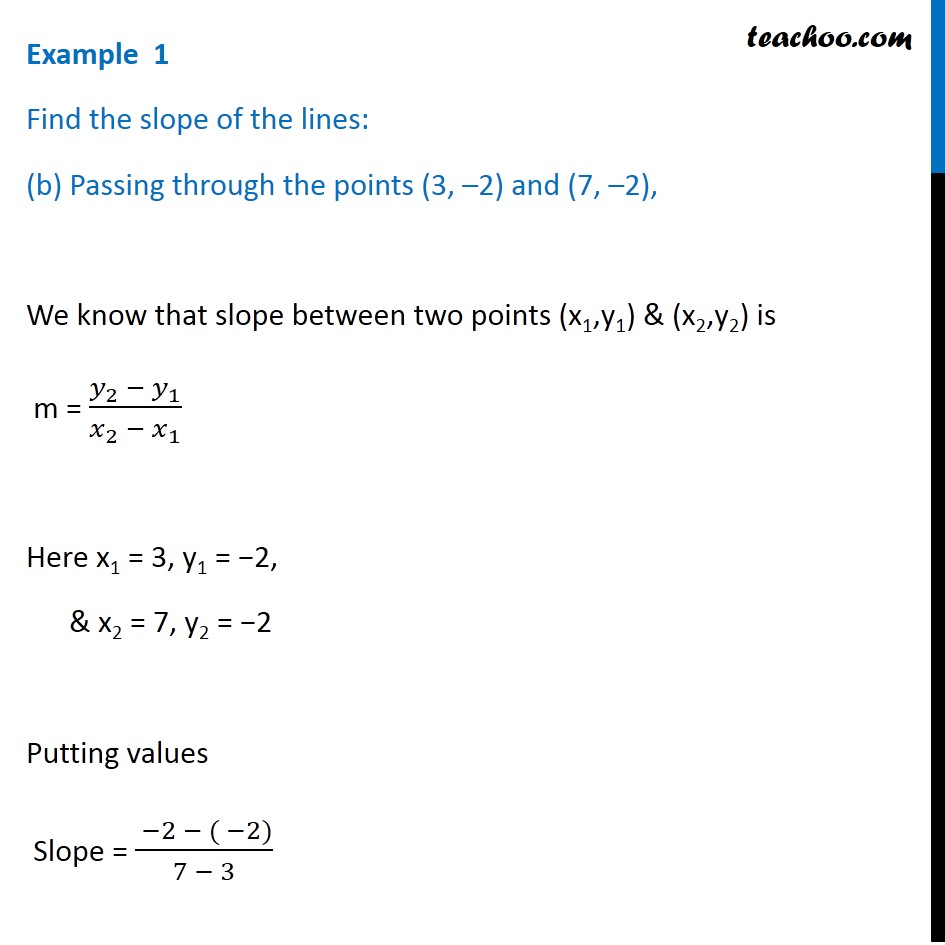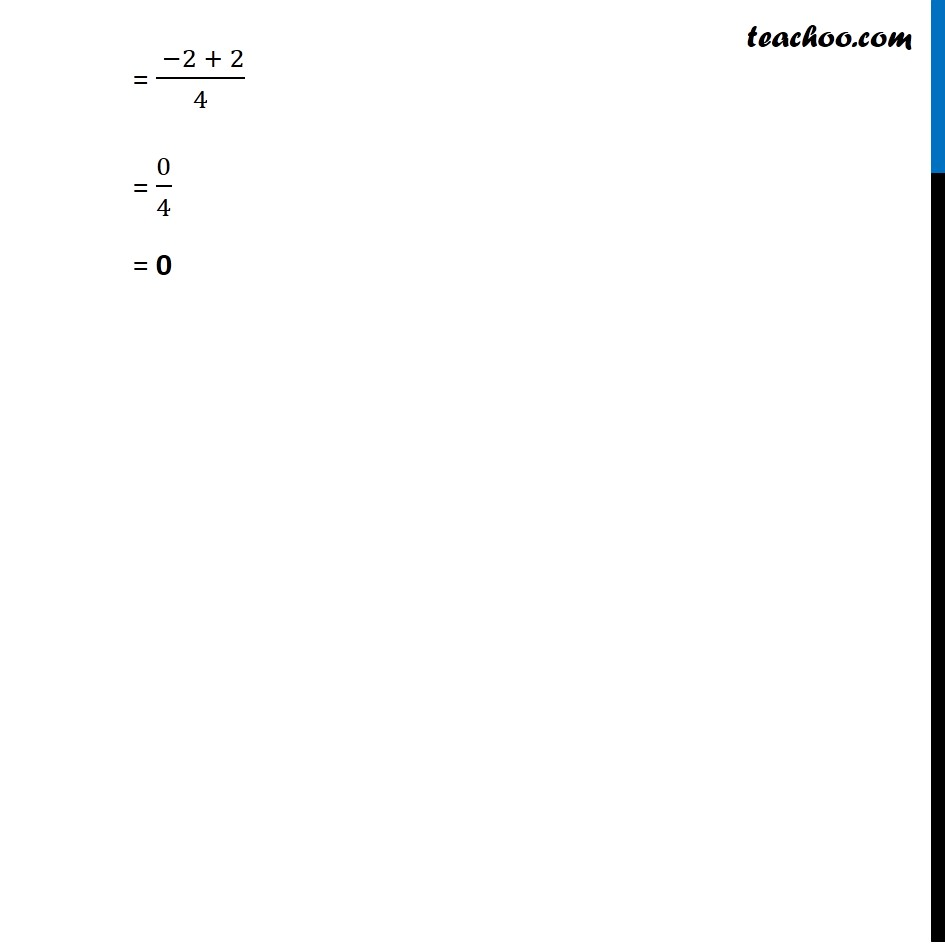Examples

Chapter 9 Class 11 Straight Lines
Serial order wiseLearn in your speed, with individual attention - Teachoo Maths 1-on-1 Class

### Transcript

Example 1 Find the slope of the lines: (b) Passing through the points (3, –2) and (7, –2), We know that slope between two points (x1,y1) & (x2,y2) is m = (𝑦_2 − 𝑦_1)/(𝑥_2 − 𝑥_1 ) Here x1 = 3, y1 = −2, & x2 = 7, y2 = −2 Putting values Slope = ( −2 − ( −2))/(7 − 3) = ( −2 + 2)/4 = 0/4 = 0